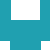#### [KS3][(i)GCSE][Homework Packs] Year 7Year 8Year 9KS 3

2020-5-2 409

Grade G
1	Place value – 1
2	Place value – 2
3	Approximation
4	Decimals: Money – 1
5	Decimals: Money – 2
6	Money: Using a calculator – 1
7	Money: Using a calculator – 2
8	Negative numbers – 1
9	Negative numbers – 2
11	Tables
12	Whole number problems with remainders
13	Calculator skills
14	Addition and subtraction – 1
15	Addition and subtraction – 2
16	2-D and 3-D shapes – 1
17	2-D and 3-D shapes – 2
18	Reflective symmetry – 1
19	Reflective symmetry – 2
20	Measures – 1
21	Measures – 2
22	Time – 2
23	Time – 2
24	Tables and lists – 1
25	Tables and lists – 2
26	Bar charts – 1
27	Bar charts – 2
28	Pictograms – 1
29	Pictograms – 2
30	Pictograms, bar charts and tables

Grade F
1	Place value
2	Multiplication facts
3	Solving problems without a calculator
4	Addition and subtraction of decimals
5	Calculation checks
6	Fractions and percentages – 1
7	Fractions and percentages – 2
8	Number patterns – 1
9	Number patterns – 2
10	Number patterns – 3
11	Using formulae
12	Co-ordinates
13	3-D models – 1
14	3-D models – 2
15	3-D models – 3
16	Common 2-D shapes
17	Congruent shapes
18	Rotational symmetry
19	Reflection – 1
20	Reflection – 2
21	Measurement – 1
22	Measurement – 2
23	Time – 1
24	Time – 2
25	Perimeter, area and volume
26	Frequency tables
27	Frequency diagrams
28	Median and mode
29	Line graphs
30	Probability

Grade E
1	Multiplying and dividing whole numbers by 10, 100 and 1000 – 1
2	Multiplying and dividing decimals by 10, 100 and 1000 – 2
3	Multiplying and dividing decimals by 10, 100 and 1000 – 3
4	Ordering, adding and subtracting negative numbers
5	Addition, subtraction, multiplication and division of decimals
6	Calculating fractional and percentage parts – 1
7	Calculating fractional and percentage parts – 2
8	Long multiplication and division without a calculator
9	Checking and estimating – 1
10	Checking and estimating – 2
11	Writing in algebra
12	Using algebra – 1
13	Using algebra – 2
14	Using a protractor
15	Angles
16	Symmetry of 2-D shapes – 1
17	Symmetry of 2-D shapes – 2
18	Rough metric equivalents of imperial units
19	Converting one metric unit to another
20	Making sensible estimates
21	Mean and range – 1
22	Mean and range – 2
23	Comparing two sets of data
24	Using and drawing conclusions from graphs – 1
25	Using and drawing conclusions from graphs – 2
26	Understanding pie charts – 1
27	Understanding pie charts – 2
28	The probability scale – 1
29	The probability scale – 2
30	Justifying probabilities

Grade D
1	Fractions – 1
2	Fractions – 2
3	Percentages – 1
4	Percentages – 2
5	Fractions, decimals and percentages – 1
6	Fractions, decimals and percentages – 2
7	Fractions, decimals and percentages – 3
8	Fractions, decimals and percentages – 4
9	Ratio – 1
10	Ratio – 2
11	Ratio – 3
12	Scale
13	Number patterns
14	Rules: Equations
15	Writing equations
16	Trial and improvement – 1
17	Trial and improvement – 2
18	Drawing lines and graphs
19	Intersecting and parallel lines
20	Regular polygons
21	Bearings
22	2-D representation of 3-D shapes
23	Properties of quadrilaterals and triangles
24	Enlargement
25	Areas and volumes
26	Circumference and area of a circle
27	Frequency tables and frequency graphs
28	Scatter diagrams
29	Drawing pie charts
30	Probability

Grade C
1	Multiplication and division by numbers less than one
2	Mental arithmetic shortcuts – 1
3	Mental arithmetic shortcuts – 2
4	Rounding numbers
5	Estimating
6	Using a calculator (brackets and memory) – 1
7	Using a calculator (using the memory) – 2
8	Solving problems
9	Fractions and percentages
10	Number patterns – 1
11	Number patterns – 2
12	Simultaneous equations
13	Solving simultaneous equations by drawing graphs
14	Inequalities – 1
15	Inequalities – 2
16	Enlargement by a fractional scale factor
17	Accuracy of measurement
18	Compound units
19	Calculating length, area and volume – 1
20	Calculating length, area and volume – 2
21	Calculating length, area and volume – 3
22	Pythagoras' theorem – 1
23	Pythagoras' theorem – 2
24	Locus
25	Designing questionnaires
26	Hypothesis
27	Comparing data
28	Grouped data
29	Scatter diagrams (line of best fit)
30	Estimation of probability by experiment

Grade B
1	Using a calculator (powers, roots and memory)
2	Standard form
3	Percentages and fractions – 1
4	Percentages and fractions – 2
5	Percentages and fractions – 3
6	Percentages and fractions – 4
7	Using algebraic formula – 1
8	Using algebraic formula – 2
9	Re-writing formulae – 1
10	Re-writing formulae – 2
11	Equations – 1
12	Equations – 2
13	Indices (powers) – 1
14	Indices (powers) – 2
15	Expansion of brackets – 1
16	Expansion of brackets – 2
17	Factorisation – 1
18	Factorisation – 2
19	Solving quadratic equations by factorisation
20	Inequalities
21	The straight line equation y = mx + c
22	Drawing graphs
23	Speed, time and distance graphs
24	Similarity
25	Formulae for length, area and volume
26	Trigonometry: Finding an angle
27	Trigonometry: Finding a side
28	Using cumulative frequency diagrams to compare distributions
29	Cumulative frequency
30	Probability